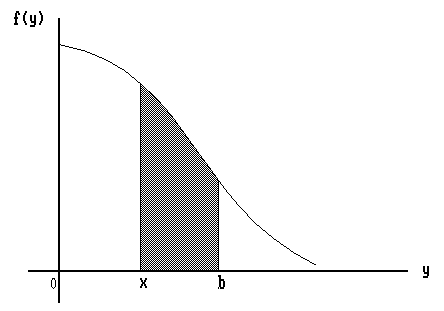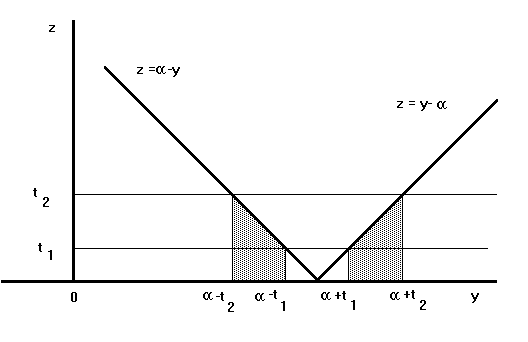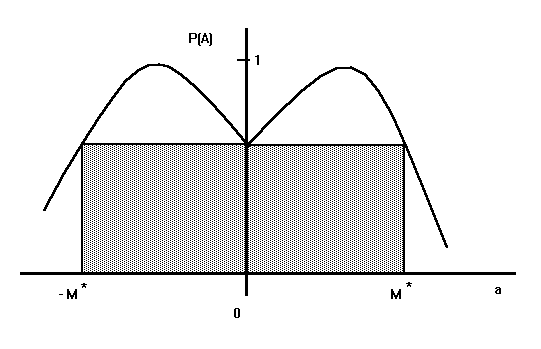# Confidence Intervals From One Observation## Abstract

Robert Machol's surprising result, that from a single observation it is possible to have finite length confidence intervals for the parameters of location-scale models, is re-produced and extended. Two previously unpublished modifications are included. First, Herbert Robbins nonparametric confidence interval is obtained. Second, I introduce a technique for obtaining confidence intervals for the scale parameter of finite length in the logarithmic metric.

## 1  Introduction

Let x be an observation from a N(m,s2) population with unknown parameters. The following statement belongs to the folklore of Statistical Science:

From a single observation x we can not gain information about the variability in the population. Thus, finite length confidence intervals for m and/or s are impossible even in principle.

This is not correct. For example xÝ|x| will cover m at least 90% of the time and (0,17|x|) will cover s at least 95% of the time. If you don't believe it check it with your PC!

I first heard about this some years ago from Herbert Robbins. According to Robbins, this phenomenon was discovered by an electrical engineer in the 60's (Robert Machol IEEE Trans. Info. Theor., 1964) but it is still relatively unknown to statisticians.

I show Machol's idea below. The intervals for m in the parametric case are due to him. The nonparametric improvement is due to Robbins and the intervals on s are mine.

## 2  Confidence Intervals for m, Parametric Case

Consider the following problem. Given a single observation from a r.v.

 X with pdf 1 s ·f( x-m s ), meIR, s > 0 unknown,
with f a known density symmetric about zero. Find a finite length 100·(1-b)% CI for m.

Machol's answer: Consider the event

 A = [ |X - m| > t |X - a| ]
where aeIR is an arbitrary constant and t > 1 is given. We have
 A = [ |Y| > t |Y - a| ]
where

 Y = X - m s with pdf f(y)  and   a = a - m s eIR.
The event A corresponds to the shaded piece in Fig. 1. Thus,Fig.  1. Illustration of event A

 P(A) = P[ |Y| > t |Y - a| ] = õõ µ¾ [(at)/(t-1)] [(at)/(t+1)] f(y) dy õõ = b(a,t)
and
 P(A) È b*(t) = sup aeIR b(a,t).
Therefore
 P[ X - t|X - a| È m È X + t|X - a| ] = P(Ac) ° 1 - b*(t)
Hence, provided that b*(t) Û 0 as t Û Ë the interval X Ýt|X-a| can be made to have any pre-specified confidence.

Example: Take f(y) = f(y) ¤  pdf of  N(0,1). From the symmetry of f about zero we can write

 b(-a,t) = õõ µ¾ -[(at)/(t-1)] -[(at)/(t+1)] f(z) dz õõ = b(a,t)
Thus,
 b*(t) = sup a > 0 b(a,t).
For a > 0 we have,
 Ñb Ña (a,t) = t t-1 f ÌÓ Ò at t-1 —¼ ½ - t t+1 f ÌÓ Ò at t+1 —¼ ½ = 0,
so that
 exp Õõ Š 1 2 ÌÓ Ò at t+1 —¼ ½ 2 - 1 2 ÌÓ Ò at t+1 —¼ ½ 2 ªº « = t+1 t-1
and taking logs we obtain
 a2 t2 (t2-1)2 [(t2+2t+1)-(t2-2t+1)] = 2 log ÌÓ Ò t+1 t-1 —¼ ½
from where
 a* = t2-1 t ÌÓ Ò 1 2t log ÌÓ Ò t+1 t-1 —¼ ½ —¼ ½ 1/2
and
 b*(t) = µ¾ U L f(y) dy
where the lower and upper limits of integration are given by:
L = (t-1)   Ì
ø

 1 2t log([(t+1)/(t-1)])

and
U = (t+1)   Ì
ø

 1 2t log([(t+1)/(t-1)])

with a calculator and a normal table we find that for t = 5 then a* = 1.0796, b* = .1 and the confidence is 90% for xÝ5|x|. Other intervals could be computed in a similar way. In fact this shows that
 P[ X - 5|X - a| È  m  È  X + 5|X - a| ]  >  .90
for all aeIR, meIR and s > 0.

The best a is the one that produces the shortest expected length. But, length = L = 2t|X - a| and

 E(L) = 2 t E(|X - a|) ç  E(|X - a|)
so that the best a = a* should minimize E(|X - a|) i.e. a* must be the median of X and since X is symmetric about m we have a* = m. Hence, the best a is our best a priori guess for m. This looks like Bayesianism sneaking in classical confidence intervals!.

The arbitrariness of a in the statement "xÝt|x -a| is a (1 - b*(t))100% CI for m" reminds me of the Stein shrinking phenomenon. Perhaps this is part of the reason why Robbins got interested in it. Recall that Robbins' Empirical Bayesianism produces Stein's estimators as a special case.

## 3  Confidence Intervals for m, Non-parametric Case

Let ê be the class of all unimodal, symmetric about zero densities. Given a single observation of X with X with pdf f(x - m) where both f eê and meIR  are unknown, find a 100(1-b)% CI for m of finite length.

Robbins' Answer: Consider first the following simple lemma:

Lemma: If f in (0,+Ë) then

 l(x) = 1 b-x µ¾ b x f(y) dy ₤ in  (0,b)
proof: This is obvious from the picture (see Fig. 2.), since l(x) denotes the mean value of f on (x,b).Fig.  2. The mean value of f(y) decreases when x approaches b

Of course the algebra gives the same answer. Notice that

 l(x)  È 1 b - x f(x) (b - x)   =  f(x).
Thus, differentiating both sides of the equation
 (b - x) l(x)  = µ¾ b x f(y) dy ,
we obtain
 lÂ(x)  = 1 b - x [ l(x) - f(x) ]  È  0
i.e. l(x) decreases in (0,b) ñ

Consider as before the event

 A = [ |X - m| > t|X - a| ] for t > 1 and aeIR.
Then, if Y = X - m , we have

 P(A)  =  P[ |Y| > t |Y - a| ] with a = a - m eIR.
 P(A)  =  b(a,t) = b(-a,t) since f eê.
But now applying the Lemma for x = at / (t+1) > 0 and b = at/ (t-1) we obtain
l(x) = P(A)
 at ÌÓ Ò 1 t-1 - 1 t+1 —¼ ½
È l(0) = t-1
at
µ
¾
at/(t-1)

0
f(y) dy È t-1
2at
.
Hence,
 P(A)  È 1 t + 1 for all  aeIR and  f eê.
Therefore
 P[ X - t|X - a|  È  m  È  X + t|X - a| ]  °  1 - 1 1+t
holds for all aeIR, meIR, and feê.

Example: For t = 9, we have 1 - 1/(1+t) = .9, and xÝ9|x-a|  will cover m at least 90% of the time even if we are uncertain about feê. This suggests the following game: Each time you pick up a function f in ê in any way you want i.e. deterministically or stochastically with some distribution. Then you choose meIR  also in an arbitrary way i.e. each m  every time or following a pre-specified sequence, or generate them with a distribution changing the distribution each time etc... Then use the computer to show me x with pdf f(x-m) . I win \$1 if xÝ9|x|  covers your m  and you win \$5 if it doesn't. Do you want to play a couple of hundred times?

## 4  Confidence Intervals for s

We consider now the estimation of the scale parameter from a single observation. It should be noticed that the only interesting confidence intervals are those of finite length. Thus, (0,Ë) is a 100% confidence interval but useless.

The natural, invariant under re-parameterizations, measure of length for a confidence interval (a,b) for a scale parameter is not just b-a but proportional to the difference in the logarithmic scale, i.e. logb - loga. This follows by recalling the fact that the square of the element of length, on the hypothesis space of the location-scale model, along a line of constant **location** (notice the typo in the original paper) is given by:

 ds2 = gss (ds)2
where gss is the Fisher information amount at s given by:

 gss = k-1 s2
with

 k = 4 µ¾ Ë -Ë y2 ( yÂ(y) )2 dy
and y2 = f in the notation of the proposition below. Hence, the geodesic distance from the probability distribution with scale ``a'' to the probability distribution with scale ``b'' is obtained by integrating the element of length and therefore proportional to the difference in the log scale as noted above. The reader unfamiliar with the geometry of hypothesis spaces may use the expression of the Kullback number between the gaussian with mean zero and standard deviation ``a'' and the gaussian with mean zero and standard deviation ``b'' as an approximation to the geodesic distance, to convince him/herself of the logarithmic nature of this length.

It is therefore necessary to consider confidence intervals with non-zero lower bounds, since s = 0 is in fact a line at infinity. I show below that it is possible to have finite length confidence intervals for the scale parameter from a single observation, but only if we rule out a priori from the hypothesis space a bit more than the line s = 0. It is this interplay between geometry, classical inference and bayesianism that I find appealing in this problem.

Proposition: Let f be a pdf symmetric about 0 and differentiable everywhere. Let F be the associated cdf. Let 0 < t1 < t2 È Ë  with fÂ(t1) > fÂ(t2)  and define

 G(a,t1,t2)  =  F(a- t1) + F(a+ t2) - F(a- t2) - F(a+ t1).
Let M > 0, aeIR, meIR, s > 0  be given numbers. Then if
 |m- a|  È  sM and  X with pdf 1 s f ÌÓ Ò x-m s —¼ ½ ,
we have
 P Õõ Š |X - a| t2 È  s  È |X - a| t1 ªº « °  2 [F(t2) - F(t1)] I[M È M*] +
 I[M > M*] inf 0 < a < M šÚ Ÿ G(a,t1,t2) ■» ± .
Where M* = min { a > 0 : G(a,t1,t2)  =  G(0,t1,t2) }. If f ¤ N(0,1)  (or any other pdf with similar tails) and excellent approximation is
 M*  =  t2 + F-1(2F(t1) - 1)
Proof: Consider the event
 A  = Õõ Š |X - a| t2 È  s  È |X - a| t1 ªº « .
Let
 Y  = X - m s with pdf  f(y).
Then by adding and subtracting m inside the absolute values and dividing through by s we obtain
 A  =   [t1  È  |Y - a|  È  t2]
where a = (a-m)/s  is such that |a|  È  M. Notice that the y's satisfying the inequalities that define the event A correspond to the shaded region in Fig. 3.Fig.  3. Illustration of event A

Hence,

 P(A)  = µ¾ a- t1 a- t2 f(y) dy + µ¾ a+ t2 a+ t1 f(y) dy  =  G(a,t1,t2)
Notice that for given values t1 and t2 the function G, as a function of a is twice differentiable and symmetric about zero with a local minimum at a = 0. Since, using the fact that f(y) = f(-y) we have

 ÑG Ña õõ õ a = 0 =  [f(a- t1) - f(a- t2) + f(a+ t2) - f(a+ t1)]|a = 0  =  0
and also
 Ñ2 G Ña2 õõ õ a = 0 =  fÂ(-t1) - fÂ(-t2) + fÂ(t2) - fÂ(t1)
 =  2 (fÂ(t1) - fÂ(t2))  >  0
Thus,
 P(A)  °  G(0,t1,t2)  =  2 [F(t2) - F(t1)]
provided that |a| È M* i.e. if M È M*. The picture (see Fig. 4.) illustrates the situation.Fig.  4. Illustration of the event A

In the gaussian case, to obtain reasonable confidences we must have t1 < 1 and t2 > 3. Hence, F(a- t1£  F(a+ t1£  F(a) and F(a+ t2£  1. From where

 G(a,t1,t2)  £  1 - F(a- t2)  ¤  2 [1 - F(t1)]  £  G(0,t1,t2)
and the approximation for M* is obtained by solving the central identity for añ

Remarks:

1) Notice that the lower bound of the confidence interval, i.e. |x - a|/ t2, is positive only if M < Ë i.e. if we know a priori that |m- a| È sM < Ë.

2) When t2 Û Ë then M* Û Ë and with no prior knowledge ( i.e. |m- a| < Ë ) we still have

 P ÌÓ Ò 0 È s È |X - a| t1 —¼ ½ °  2 (1 - F(t1)).

3) The value of t2 is related to the amount of prior information. The larger t2 the weaker the prior information necessary to assume the desire confidence. On the other hand t1 controls the confidence associated to the interval. These remarks are illustrated with examples.

Examples: Let x be a single observation from a gaussian with unknown mean m and unknown variance s2. Then 90% CIs for s are:

(0,8|x|)    valid always

([(|x|)/4], 8 |x| )    valid if |m| È 2.7s

([(|x|)/8], 8 |x| )    valid if |m| È 6.7s

95% CIs are:

([(|x|)/5], 17|x| )    valid if |m| È 3.3s

([(|x|)/50],17|x| )    valid if |m| È 48s

(0 , 17 |x| )      valid always.

99% CIs are:

([(|x|)/5],70|x| )    valid if |m| È 2.7s

([(|x|)/(103)],70|x|)   valid if |m| È 997s

(0,70|x|)    valid always.

## Almost Real Example

I'll try to show that the required prior knowledge necessary to have non-zero lower bounds for the CIs is in fact often available. Suppose that we want to measure the length of the desk in my office with a regular meter graduated in centimeters. Let x be the result of a single measurement and let m be the true length of my desk. Then
 x = m+ e with  e  with pdf  N(0,s2)
is a reasonable and very popular assumption. Now, even before I make the measurement I can write with all confidence that for my desk

m = 2 Ý1m i.e. |m- 2| È 1. With the meter graduated in centimeters I will be guessing the middle line between centimeters so I can be sure that x = mÝ at least [1/ 4] of a centimeter. Thus,

 3 s ° 1 400 .
Therefore I can be absolutely sure that
 |m- 2|  È  1200 s.
Hence,
 ÌÓ Ò |x - 2| 1500 , 70 |x - 2| —¼ ½
will be a 99% CI for s.

File translated from TEX by TTH, version 1.94.
On 13 Oct 1998, 14:40.